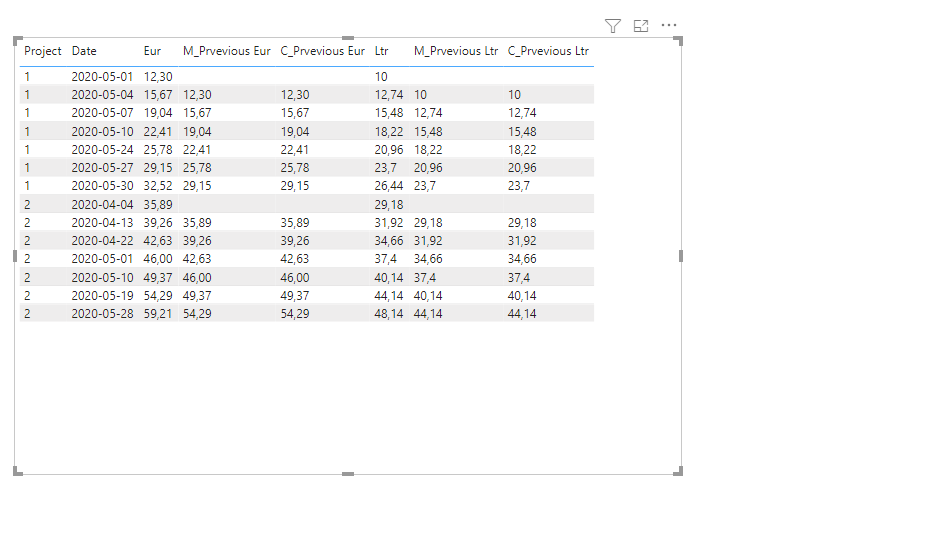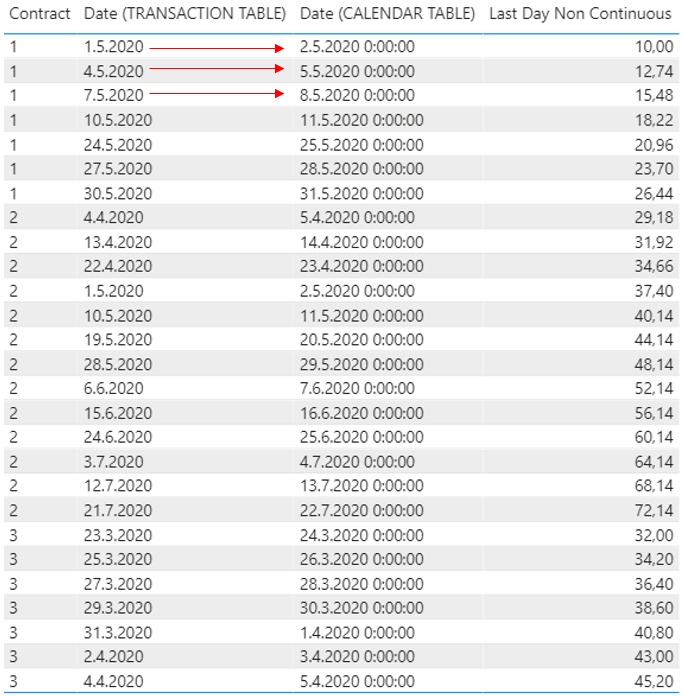cancel
Showing results for
Did you mean:Frequent Visitor

## Values from previous transaction date

Hi,

I am trying to create DAX measures to get values from previous transaction dates. On left side is Transactions table where is should be able to calculate LTR and EUR values from previous transaction dates. My goal is on right side where I have marked with colours the results which I would like to get ("Previous Ltr" and "Previos EUR"). I have spent so many hours trying to get this working so it would so great if someone could help me?

In addtition this case linked to quite big dataset so I would like to know if it is better to create calculated columns for this? Or are we able to solve this with measure only?1 ACCEPTED SOLUTIONCommunity Support

Hi, @K_LLI

I prefer to  use calculated column as below:

``````C_Prvevious Eur =
CALCULATE (
MAX ( Transactions[Eur] ),
Transactions,
Transactions[Date] < EARLIER ( Transactions[Date] ),
Transactions[Project] = EARLIER ( Transactions[Project] )
)
``````
``````C_Prvevious Ltr =
CALCULATE (
MAX ( Transactions[Ltr] ),
Transactions,
Transactions[Date] < EARLIER ( Transactions[Date] ),
Transactions[Project] = EARLIER ( Transactions[Project] )
)
``````

You  can also try measure as below:

``````M_Prvevious Eur =
VAR previousdate =
CALCULATE (
MAX ( Transactions[Date] ),
FILTER (
ALLEXCEPT ( Transactions, Transactions[Project] ),
Transactions[Date] < MAX ( Transactions[Date] )
)
)
RETURN
CALCULATE (
MAX ( Transactions[Eur] ),
FILTER ( ALLEXCEPT(  Transactions,Transactions[Project]), Transactions[Date] = previousdate )
)``````
``````M_Prvevious Ltr =
VAR previousdate =
CALCULATE (
MAX ( Transactions[Date] ),
FILTER (
ALLEXCEPT ( Transactions, Transactions[Project] ),
Transactions[Date] < MAX ( Transactions[Date] )
)
)
RETURN
CALCULATE (
MAX ( Transactions[Ltr] ),
FILTER ( ALLEXCEPT(  Transactions,Transactions[Project]), Transactions[Date] = previousdate )
)``````Please check my attached pbix file for more details.

Best Regards,
Community Support Team _ Eason
If this post helps, then please consider Accept it as the solution to help the other members find it more quickly.

4 REPLIES 4Community Support

Hi, @K_LLI

I prefer to  use calculated column as below:

``````C_Prvevious Eur =
CALCULATE (
MAX ( Transactions[Eur] ),
Transactions,
Transactions[Date] < EARLIER ( Transactions[Date] ),
Transactions[Project] = EARLIER ( Transactions[Project] )
)
``````
``````C_Prvevious Ltr =
CALCULATE (
MAX ( Transactions[Ltr] ),
Transactions,
Transactions[Date] < EARLIER ( Transactions[Date] ),
Transactions[Project] = EARLIER ( Transactions[Project] )
)
``````

You  can also try measure as below:

``````M_Prvevious Eur =
VAR previousdate =
CALCULATE (
MAX ( Transactions[Date] ),
FILTER (
ALLEXCEPT ( Transactions, Transactions[Project] ),
Transactions[Date] < MAX ( Transactions[Date] )
)
)
RETURN
CALCULATE (
MAX ( Transactions[Eur] ),
FILTER ( ALLEXCEPT(  Transactions,Transactions[Project]), Transactions[Date] = previousdate )
)``````
``````M_Prvevious Ltr =
VAR previousdate =
CALCULATE (
MAX ( Transactions[Date] ),
FILTER (
ALLEXCEPT ( Transactions, Transactions[Project] ),
Transactions[Date] < MAX ( Transactions[Date] )
)
)
RETURN
CALCULATE (
MAX ( Transactions[Ltr] ),
FILTER ( ALLEXCEPT(  Transactions,Transactions[Project]), Transactions[Date] = previousdate )
)``````Please check my attached pbix file for more details.

Best Regards,
Community Support Team _ Eason
If this post helps, then please consider Accept it as the solution to help the other members find it more quickly.Frequent Visitor

Hi,

I added date from transactions table and date from Date table to report and now it is shifting the calculation to next day (1.5.2020 -> 2.5.2020). The calculation should be shifted to next transaction date (1.5.2020 -> 4.5.2020) as shown in my first post. Can anyone help?

I have joined my calendar table to this transaction table and marked is as date table.

Thanks for the support @amitchandak!  do you find any solutions to this?Frequent Visitor

Thank you for your support! Regardin this part:

MAXX(FILTER(ALLSELECTED('Date'),'Date'[Date]<max('Date'[Date])),'Date'[Date])))

Should this refer to transactions table? Do I create this as measure or calculated column?Super User

@K_LLI , with help from a date table

Last Day Non Continuous = CALCULATE([ltr],filter(ALLSELECTED('Date'),'Date'[Date] =MAXX(FILTER(ALLSELECTED('Date'),'Date'[Date]<max('Date'[Date])),'Date'[Date])))

Last Day Non Continuous EUR = CALCULATE([Eur],filter(ALLSELECTED('Date'),'Date'[Date] =MAXX(FILTER(ALLSELECTED('Date'),'Date'[Date]<max('Date'[Date])),'Date'[Date])))

To get the best of the time intelligence function. Make sure you have a date calendar and it has been marked as the date in model view. Also, join it with the date column of your fact/s. Refer :radacad sqlbi My Video Series Appreciate your Kudos.

!! Microsoft Fabric !!
Microsoft Power BI Learning Resources, 2023 !!
Learn Power BI - Full Course with Dec-2022, with Window, Index, Offset, 100+ Topics !!
Did I answer your question? Mark my post as a solution! Appreciate your Kudos !! Proud to be a Super User! !!